## Putting Math to Work

Type of Unit: Problem Solving

# Prior Knowledge

Students should be able to:

• Solve problems involving all four operations with rational numbers.
• Write ratios and rates.
• Write and solve proportions.
• Solve problems involving scale.
• Write and solve equations to represent problem situations.
• Create and interpret maps, graphs, and diagrams.
• Use multiple representations (i.e., tables, graphs, and equations) to represent problem situations.
• Calculate area and volume.
• Solve problems involving linear measurement.

# Lesson Flow

Students apply and integrate math concepts they have previously learned to solve mathematical and real-world problems using a variety of strategies. Students have opportunities to explore four real-world situations involving problem solving in a variety of contexts, complete a project of their choice, and work through a series of Gallery problems.

First, students utilize their spatial reasoning and visualization skills to find the least number of cubes needed to construct a structure when given the front and side views. Then, students select a project to complete as they work through this unit to refine their problem-solving skills. Students explore the relationship between flapping frequency, amplitude, and cruising speed to calculate the Strouhal number of a variety of flying and swimming animals. After that, students explore the volume of the Great Lakes, applying strategies for solving volume problems and analyzing diagrams. Next, students graphically represent a virtual journey through the locks of the Welland Canal, estimating the amount of drop through each lock and the distance traveled. Students have a day in class to work on their projects with their group.

Then, students have two days to explore Gallery problems of their choosing. Finally, students present their projects to the class.

Subject:
Mathematics
Material Type:
Unit of Study
Level:
Middle School
7
Tags:
• Problem Solving
• Applied Mathematics
• Strouhal Numbers
• Volume
• Measurements and Data
• Distance
• Travel
• Visualization
• Cube Structure
Unit 7 Putting Math to Work# Education Standards

••••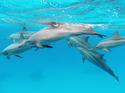•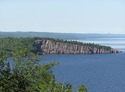•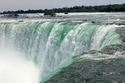•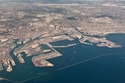•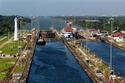•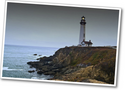••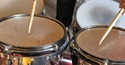•